GFG App
Open AppBrowser
Continue

# Class 9 RD Sharma Solutions – Chapter 11 Coordinate Geometry – Exercise 11.1

### Question 1. Plot the following points on the graph paper:

(i) (2, 5)      (ii) (4, -3)      (iii) (-5, -7)     (iv) (7, -4)     (v) (-3, 2)

(vi) (7, 0)    (vii) (-4, 0)    (viii) (0, 7)      (ix) (0, -4)     (x) (0, 0)

Solution:

Let us considered O (0, 0) be the origin.

(i) Let the given point be A = (2, 5)

Now, the A is at 5 units of distance from x- axis and at 2 units of distance from y-axis.

So, A is in the 1st quadrant.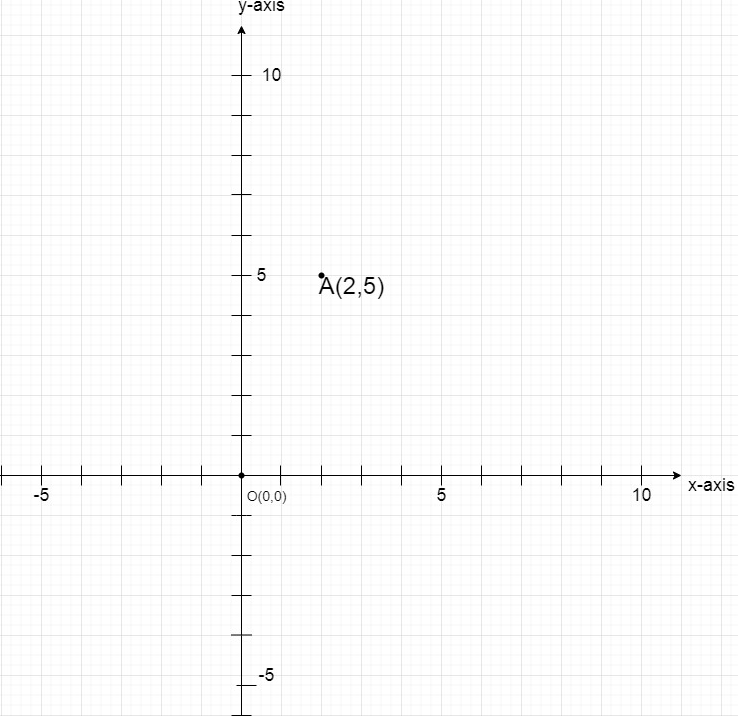(ii) Let the given point be B = (4,-3)

Now, the B is at -3 units of distance from x- axis and at 4 units of distance from y-axis.

So, B is in the 4th quadrant.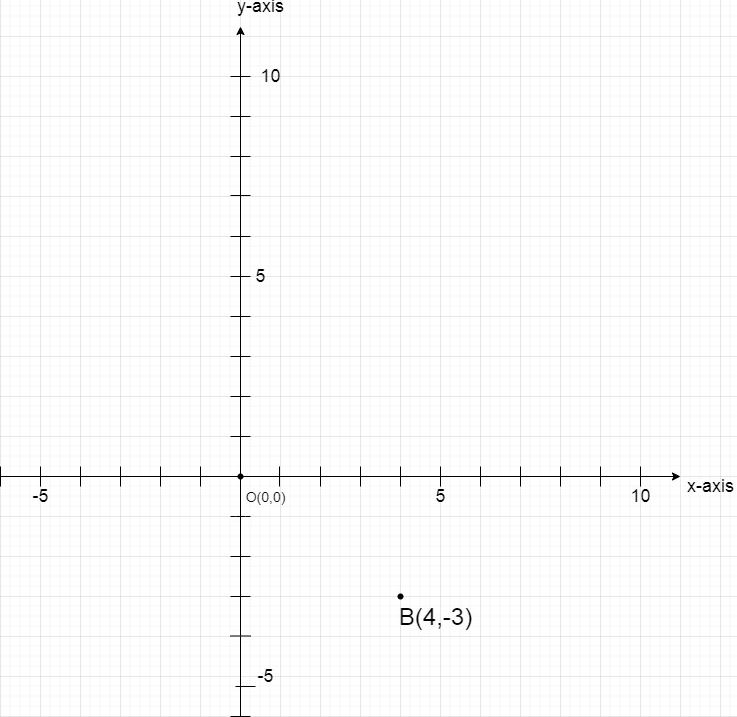(iii) Let the given point be C = (-5, -7)

Now, the C is at -7 units of distance from x- axis and at -5 units of distance from y-axis.

So, C is in the 3rd quadrant.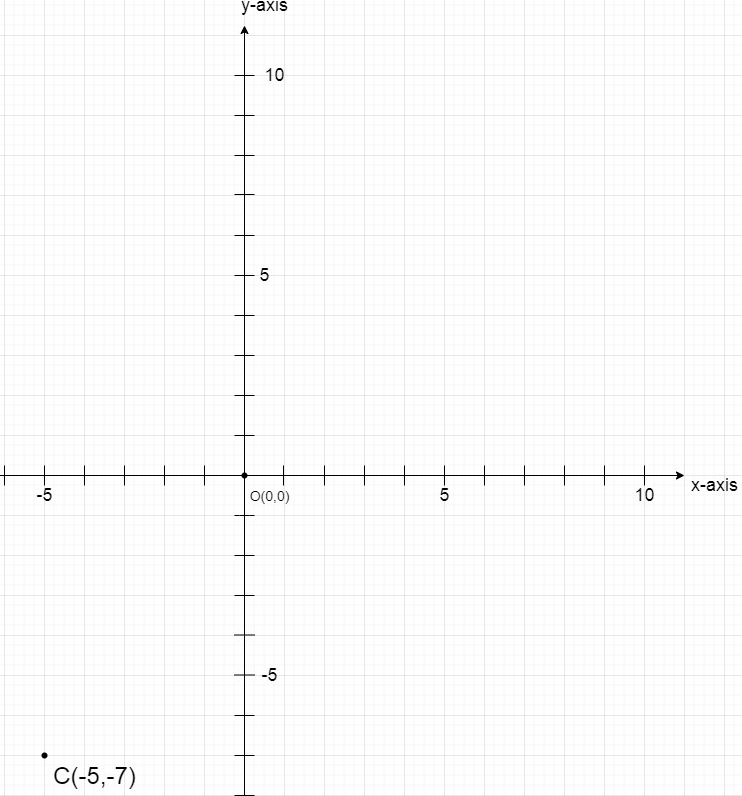(iv) Let the given point be D = (7, -4)

Now, the D is at -4 units of distance from x- axis and at 7 units of distance from y-axis.

So, D is in the 4th quadrant.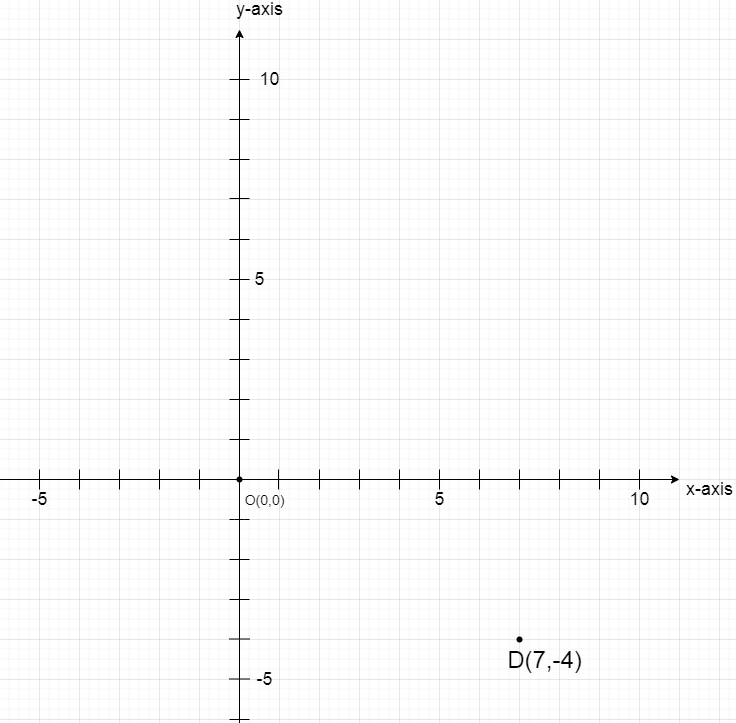(v) Let the given point be E = (-3, 2)

Now, the E is at 2 units of distance from x- axis and at -3 units of distance from y-axis.

So, E is in the 2nd quadrant.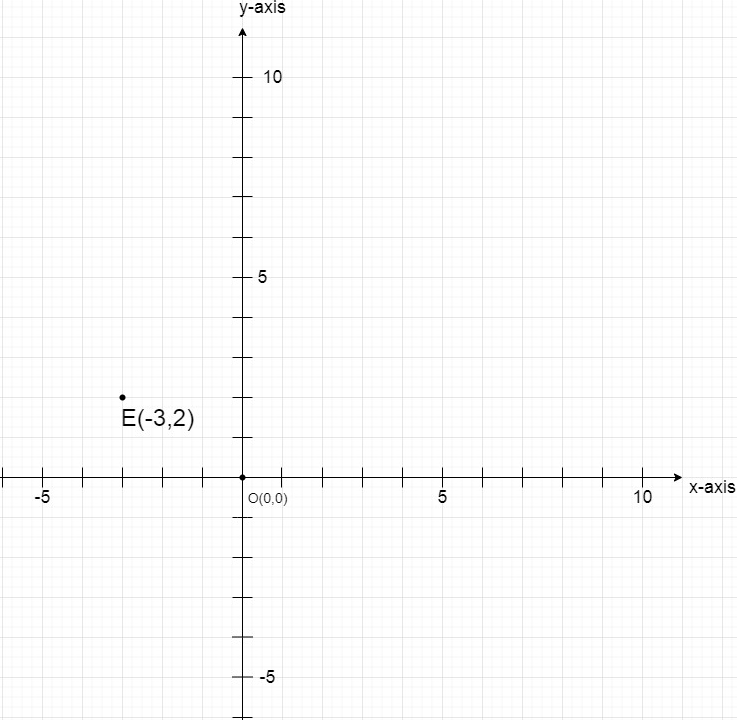(vi) Let the given point be F = (7, 0)

Now, the F is at 0 units of distance from x- axis and at 7 units of distance from y-axis.

So, F is at the positive x-axis.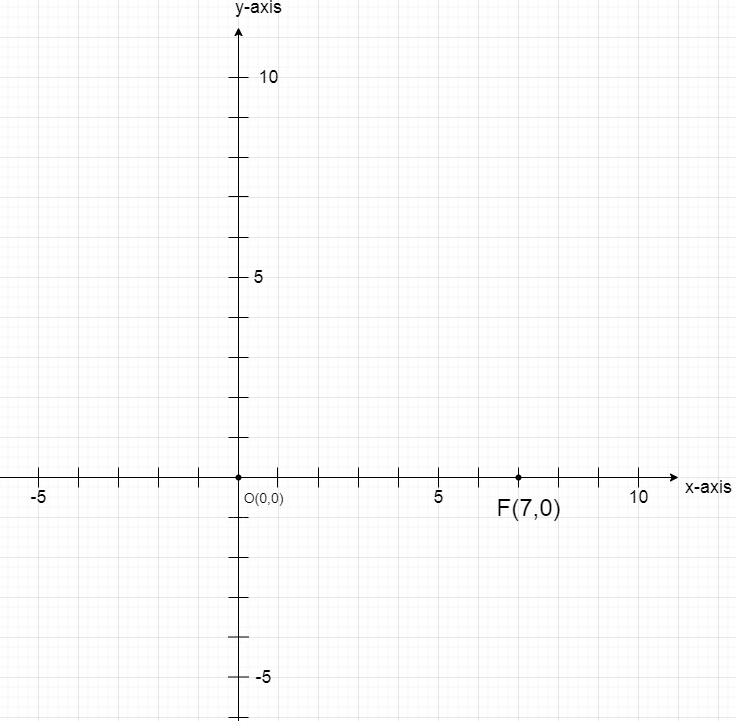(vii) Let the given point be G =(-4, 0)

Now, the G is at 0 units of distance from x- axis and at -4 units of distance from y-axis.

So, G is at the negative x-axis.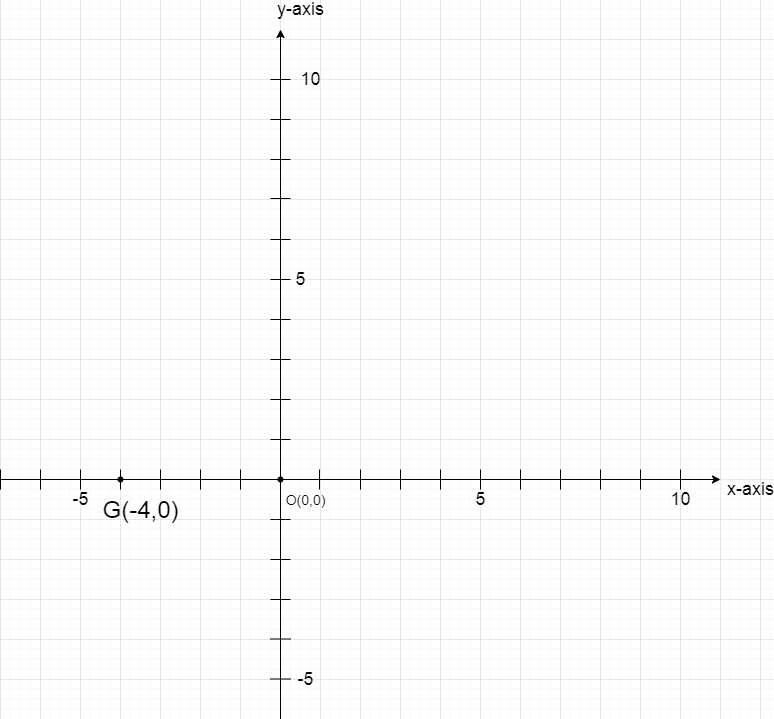(viii) Let the given point be H = (0, 7)

Now, the H is at 0 units of distance from x- axis and at 7 units of distance from y-axis.

So, H is at the positive y-axis.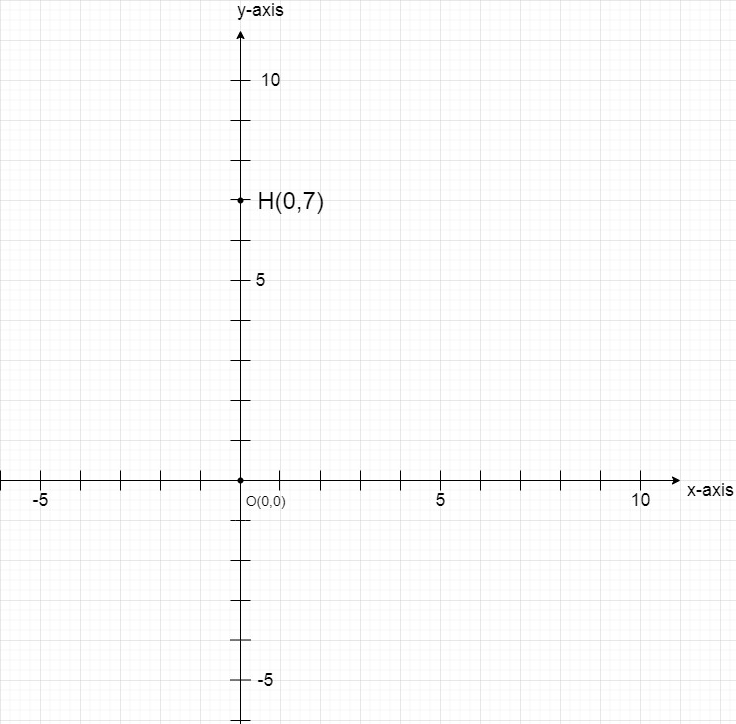(ix) Let the given point be I = (0, -4)

Now, I at -4 units of distance from x- axis and at 0 units of distance from y-axis.

So, I at the negative y-axis.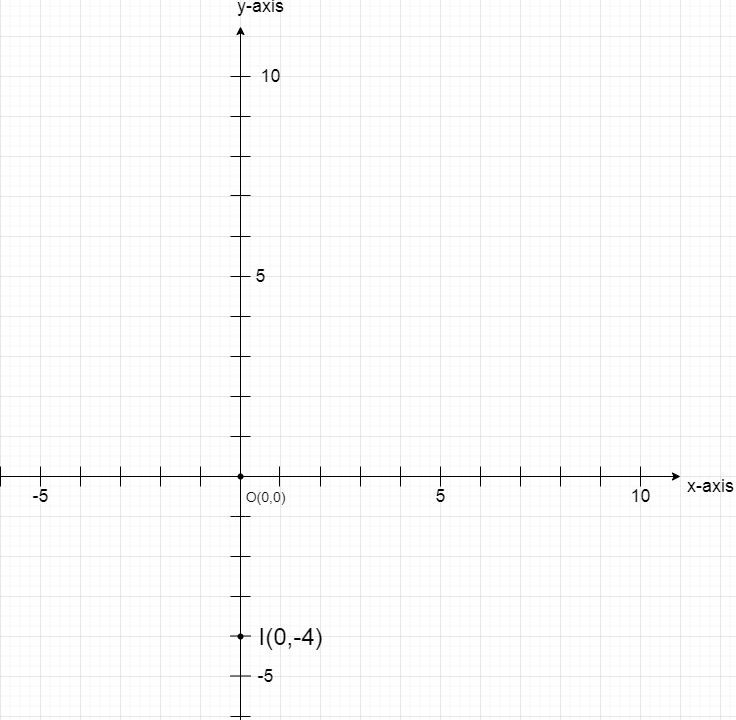(x) Let the given point be J = (0, 0)

Now, the J is at 0 units of distance from x- axis and at 0 units of distance from y-axis.

So, J is the origin only.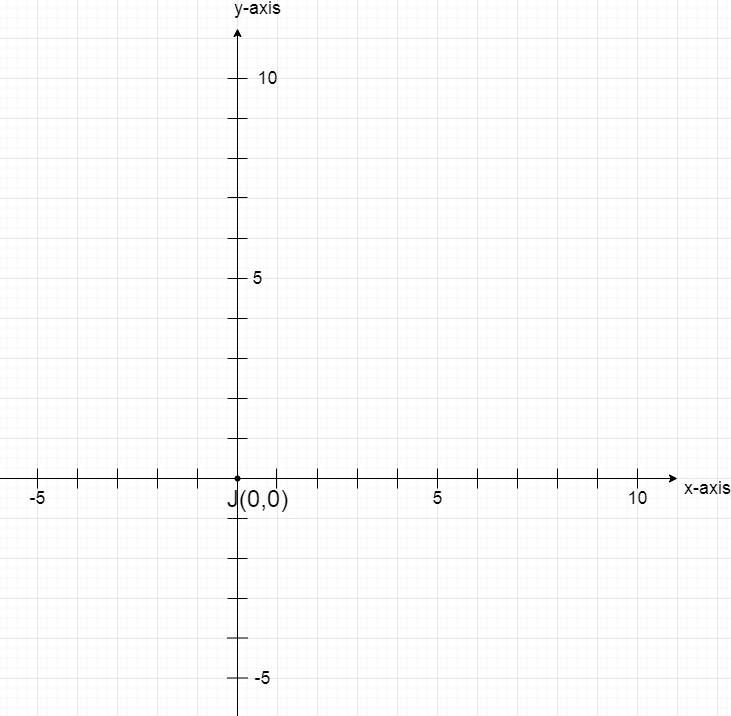### Question 2. Write the coordinates of each of the following points marked in the graph paper.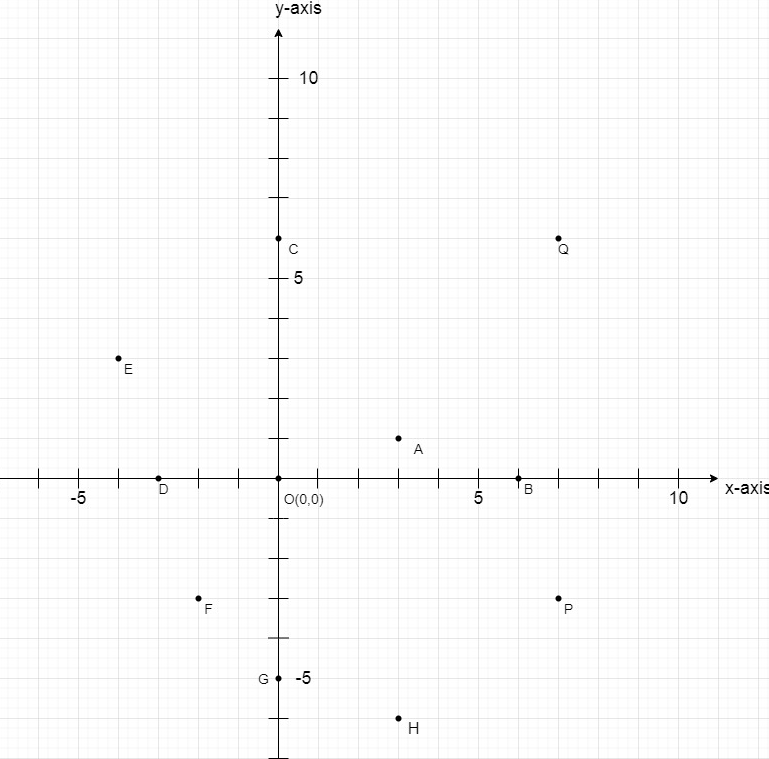Solution:

Here, Point A is at 1 units of distance from x- axis and at 3 units of distance from y-axis.

So, coordinates of point A is (3, 1).

Here, Point B is at 0 units of distance from x- axis and at 6 units of distance from y-axis.

So, coordinates of point B is (6, 0).

Here, Point C is at 6 units of distance from x- axis and at 0 units of distance from y-axis.

So, coordinates of point C is (0, 6).

Here, Point D is at 0 units of distance from x- axis and at -3 units of distance from y-axis.

So, coordinates of point D is (-3, 0).

Here, Point E is at 3 units of distance from x- axis and at -4 units of distance from y-axis.

So, coordinates of point E is (-4, 3).

Here, Point F is at -4 units of distance from x- axis and at -2 units of distance from y-axis.

So, coordinates of point F is (-2, -4).

Here, Point G is at -5 units of distance from x- axis and at 0 units of distance from y-axis.

So, coordinates of point G is (0, -5).

Here, Point H is at -6 units of distance from x- axis and at 3 units of distance from y-axis.

So, coordinates of point H is (3, -6).

Here, Point P is at -3 units of distance from x- axis and at 7 units of distance from y-axis.

So, coordinates of point P is (7, -3).

Here, Point Q is at 6 units of distance from x- axis and at 7 units of distance from y-axis.

So, coordinates of point Q is (7, 6).

Hence, all the points are A(3, 1), B(6, 0), C(0, 6), D(-3, 0), E(-4, 3), F(-2, -4), G(0, -5),

H(3, -6), P(7, -3) and Q(7, 6).

My Personal Notes arrow_drop_up